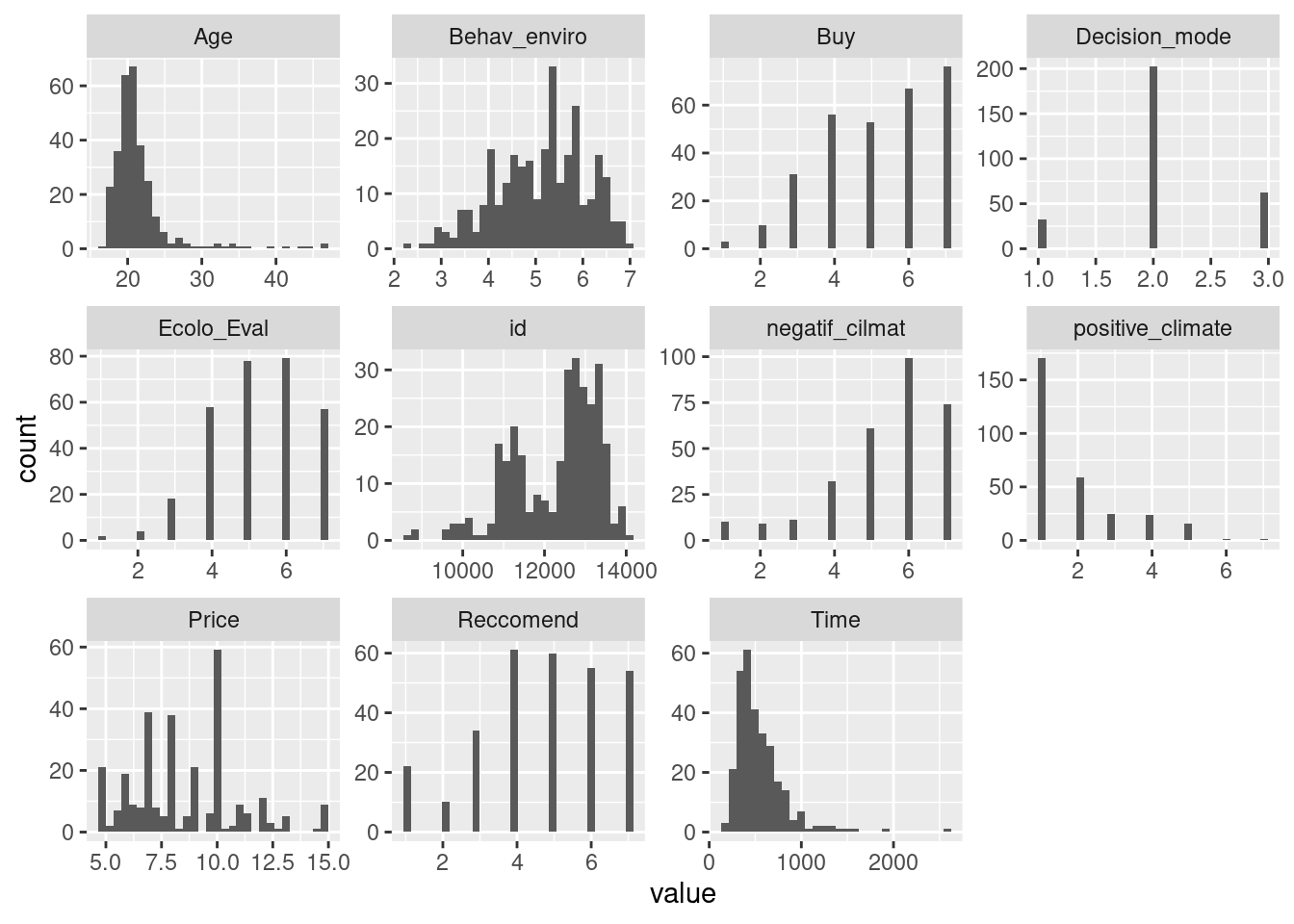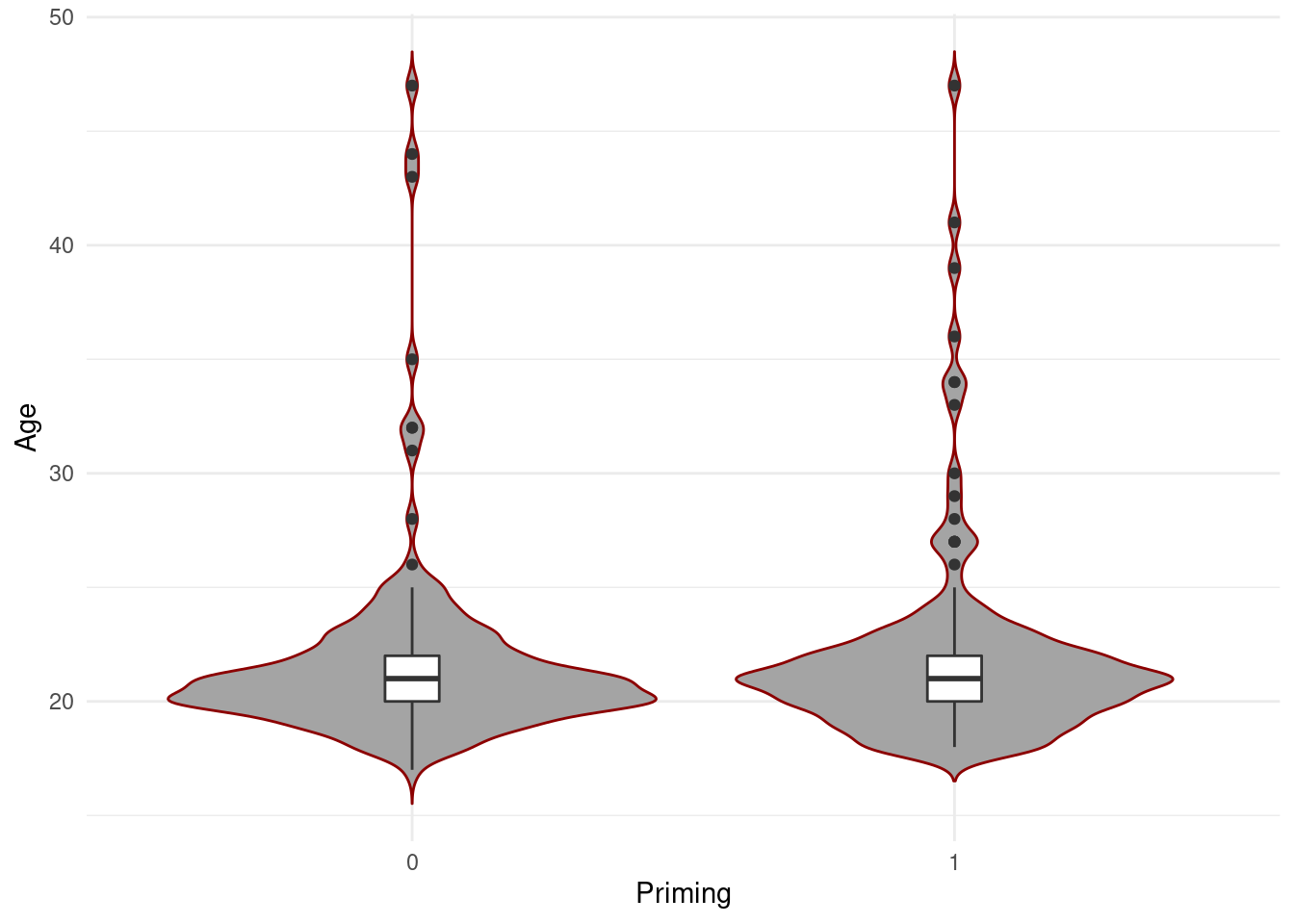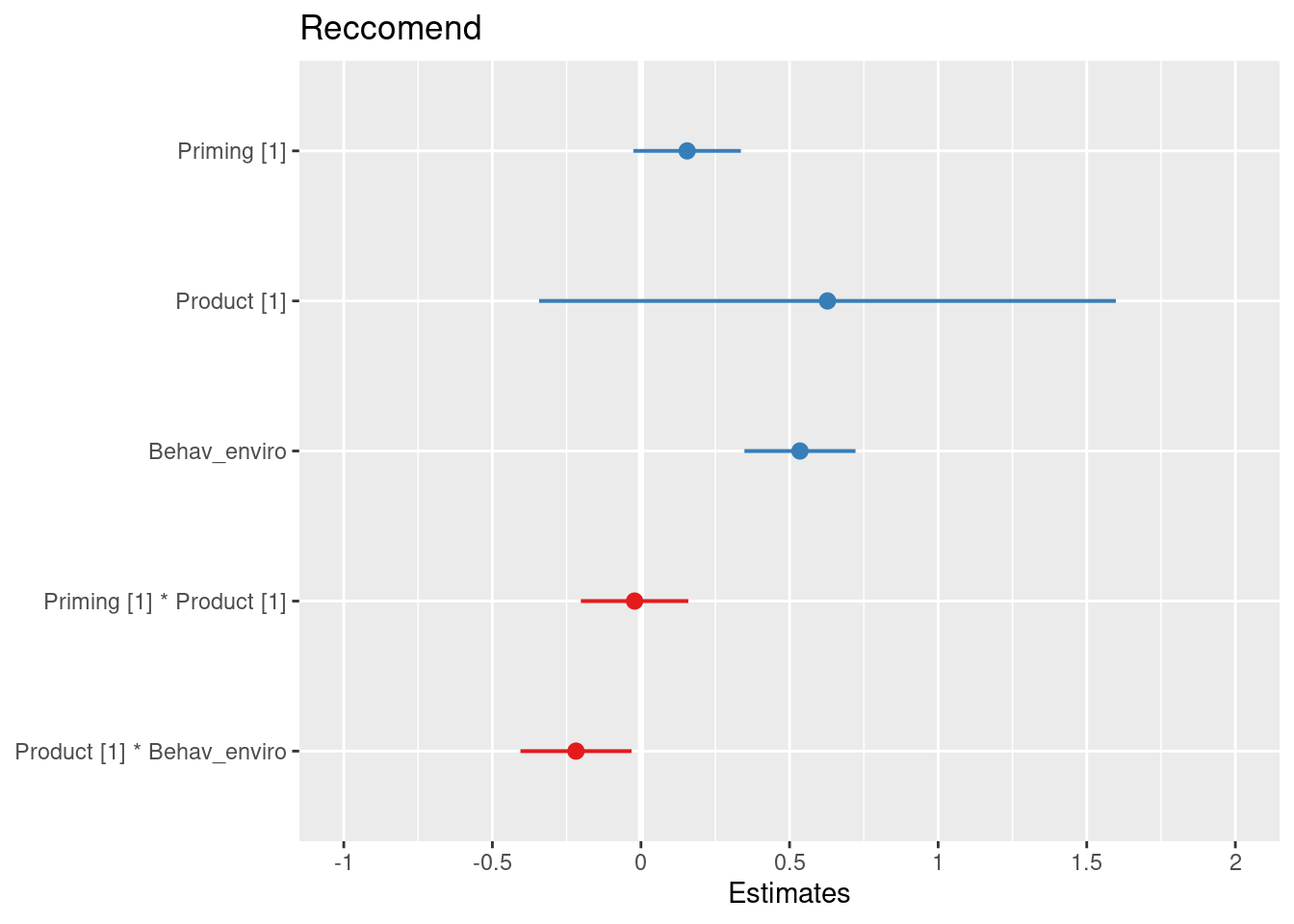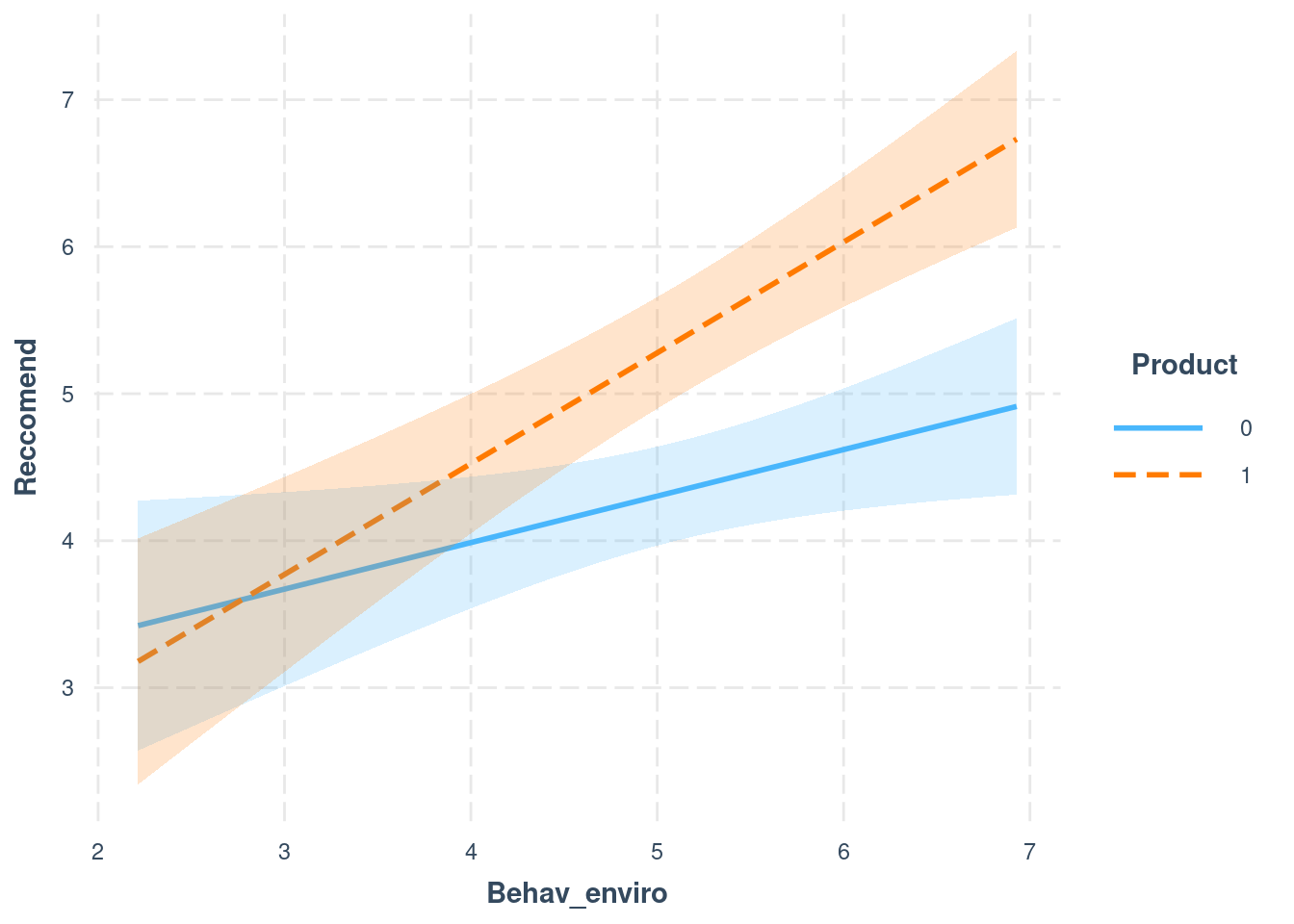### Setup

#this is to check to see if package are installed and if not to install them or just load them if they are installed!
if(!require(pacman)) {install.packages(c("pacman", "remotes"))}

if(!require(papaja)) {remotes::install_github("crsh/papaja")}

pacman::p_load(here, tinylabels, apaTables, tidyverse, gtsummary, car, GGally, MASS, rcompanion, moments, utils, sjPlot, interactions, kableExtra, report, papaja, bookdown)

#get relative path
path =  here("RESSOURCES") #this is really important ! you juste need to put the name of YOUR folder and here() will find the relative path for you !
setwd(path) #set working directory

session = utils::sessionInfo() # get session informations

#opts_chunk$set(echo = F, message=F, warning=F) # set echo F for all options(scipen = 666, warn=-1, contrasts=c("contr.sum","contr.poly"), knitr.kable.NA = '') #remove scientific notation # remove warnings #set contrasts to sum ! set.seed(666) #set random seed %notin% <- Negate(%in%) # panderOptions('knitr.auto.asis', FALSE) #remove auto styling $$~$$ $$~$$ ### Introduction Blabla citation in parentesis (James 1890) blbabla, or citation in text James (1890) $$~$$ $$~$$ #### Clean up $$~$$ $$~$$ base$Priming = as.factor(base$Priming); base$Product = as.factor(base$Product);  $$~$$ $$~$$ #### Demographics $$~$$ $$~$$ base[c("Age", "Gender", "Profession")] %>% tbl_summary(statistic = list(all_continuous() ~ "{mean} ({sd})", all_categorical() ~ "{n} / {N} ({p}%)"),) %>% modify_caption("**Table 1. Demographics **") %>%bold_labels() **Table 1. Demographics ** Characteristic N = 2961 Age 22 (4) Gender Autre/NA 2 / 296 (0.7%) Femme 239 / 296 (81%) Homme 55 / 296 (19%) Profession Actif.ve 3 / 296 (1.0%) Etudiant.e 291 / 296 (98%) Les deux 2 / 296 (0.7%) 1 Mean (SD); n / N (%) $$~$$ $$~$$ ### Inspections $$~$$ $$~$$ base %>% keep(is.numeric) %>% gather() %>% ggplot(aes(value)) + facet_wrap(~key, scales = "free") + geom_histogram()#or GGally $$~$$ $$~$$ ggplot(base, aes(x=Priming, y=Age)) + geom_violin(trim=FALSE, fill='#A4A4A4', color="darkred")+ geom_boxplot(width=0.1) + theme_minimal()$$~$$ $$~$$ ### Statistics $$~$$ $$~$$ #### Reccomendation base_recco = filter(base, id %notin% c("13594")) #remove huge outliers, see appendix final_recco = lm(Reccomend ~Priming*Product + Behav_enviro + Product:Behav_enviro, data = base_recco) apa_lm <- papaja::apa_print(anova(final_recco)) papaja::apa_table(apa_lm$table,  caption = "Anova table for Reccomendation.", label =NULL)
(#tab:Report_Regression)
Anova table for Reccomendation.
Effect $$F$$ $$\mathit{df}_1$$ $$\mathit{df}_2$$ $$\mathit{MSE}$$ $$p$$ $$\hat{\eta}^2_G$$
Priming 0.76 1 289 2.44 .383 .003
Product 32.23 1 289 2.44 < .001 .100
Behav enviro 33.11 1 289 2.44 < .001 .103
Priming $$\times$$ Product 0.06 1 289 2.44 .808 .000
Product $$\times$$ Behav enviro 5.30 1 289 2.44 .022 .018
sjPlot::plot_model(final_recco)$$~$$ $$~$$

interactions::interact_plot(final_recco, pred = Behav_enviro, modx = Product, interval = TRUE)$$~$$ $$~$$

### Conclusions

$$~$$ $$~$$

Example for reporting :

Product ($$F(1, 289) = 32.23$$, $$\mathit{MSE} = 2.44$$, $$p < .001$$, $$\hat{\eta}^2_G = .100$$) and Behav_enviro ($$F(1, 289) = 33.11$$, $$\mathit{MSE} = 2.44$$, $$p < .001$$, $$\hat{\eta}^2_G = .103$$) affected reccomendation. However, the effect of Behav_enviro differed by Product, $$F(1, 289) = 5.30$$, $$\mathit{MSE} = 2.44$$, $$p = .022$$, $$\hat{\eta}^2_G = .018$$.

$$~$$ $$~$$

### Appendix

$$~$$ $$~$$

#### Visual check normality

plotNormalHistogram(base$Reccomend, main = "Reccomendation", sub = paste("skewness =", round(skewness(base$Reccomend, na.rm = TRUE),2)))